# Estimating Correlated Constraint Boundaries from timeseries data The

• Slides: 17Estimating Correlated Constraint Boundaries from timeseries data: The multidimensional German Tank Problem Abhilasha Aswal G N S Prasanna IIIT-B EURO XXIV LisbonThe German Tank Problem n Biased estimators ¨ Maximum n likelihood Unbiased estimators ¨ Minimum Variance unbiased estimator (UMVU) ¨ Maximum Spacing estimator ¨ Bias-corrected maximum likelihood estimator EURO XXIV Lisbon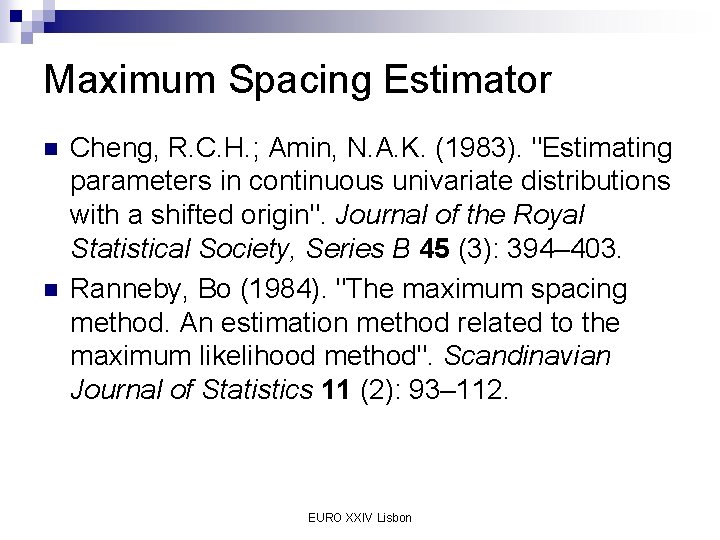Maximum Spacing Estimator n n Cheng, R. C. H. ; Amin, N. A. K. (1983). "Estimating parameters in continuous univariate distributions with a shifted origin". Journal of the Royal Statistical Society, Series B 45 (3): 394– 403. Ranneby, Bo (1984). "The maximum spacing method. An estimation method related to the maximum likelihood method". Scandinavian Journal of Statistics 11 (2): 93– 112. EURO XXIV LisbonThe General Problem n n Given correlated data samples, drawn from a uniform distribution- estimating the bounded region formed by correlated constraints enclosing the samples. Estimating the constraints without bias and with minimum variance. EURO XXIV LisbonA new UMVU for the general problem n n Generate the convex hull for the given samples. The convex hull has a very large number of facets, hence the generated convex hull facets are clustered using the following approach – ¨ Every N-dimensional facet is mapped to a point in N+1 D space as follows: All such points are K-means clustered into M clusters. ¨ The points in a cluster are replaced by a single point by taking average of all the elements. ¨ The averaged points are mapped back to the facet space forming a constrained region with fewer number of facets, approximating the convex hull. ¨ EURO XXIV LisbonA new UMVU for the general problem n Advantages ¨ Asymptotically consistent and unbiased. ¨ Fast convergence. ¨ Model independent. n A model dependent approach can be based on linear programming. EURO XXIV Lisbon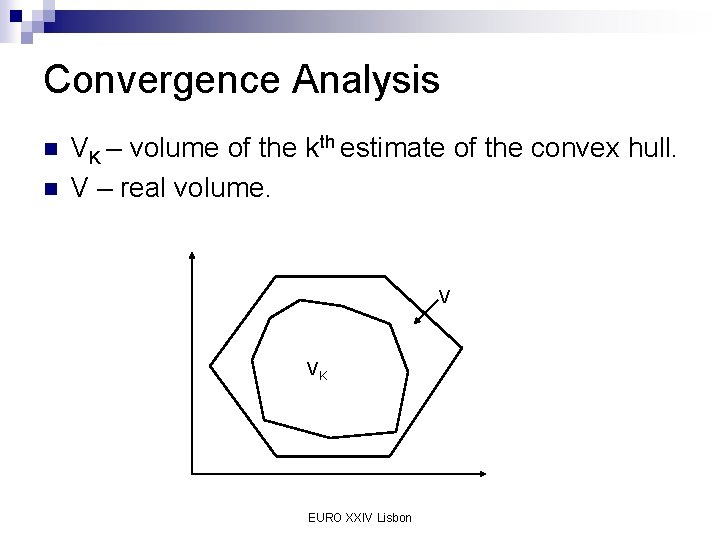Convergence Analysis n n VK – volume of the kth estimate of the convex hull. V – real volume. V VK EURO XXIV LisbonConvergence Analysis EURO XXIV Lisbon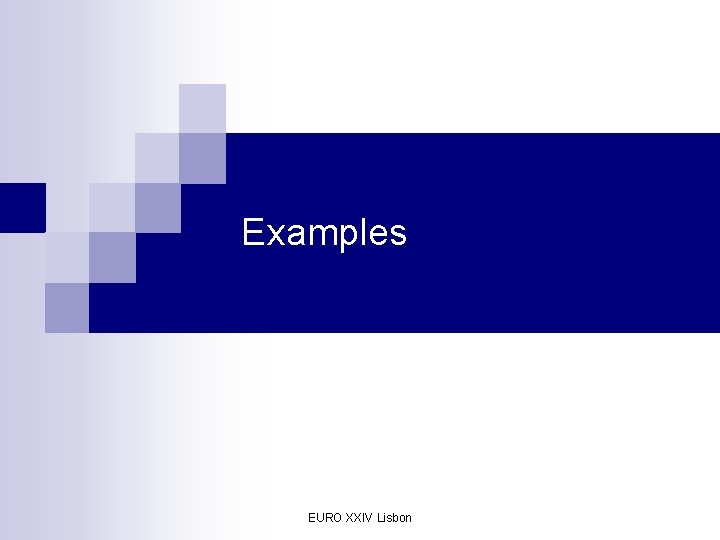Examples EURO XXIV LisbonExample 1 - A 2 D example n Constraints: x + y <= 25 ¨ x + y >= 10 ¨ x - y <= 30 ¨ x - y >= 7 ¨ n 70 samples uniformly taken EURO XXIV Lisbon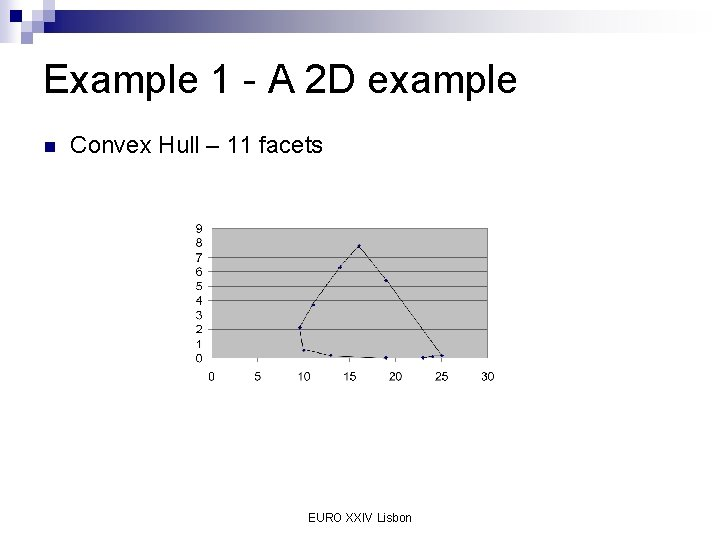Example 1 - A 2 D example n Convex Hull – 11 facets EURO XXIV Lisbon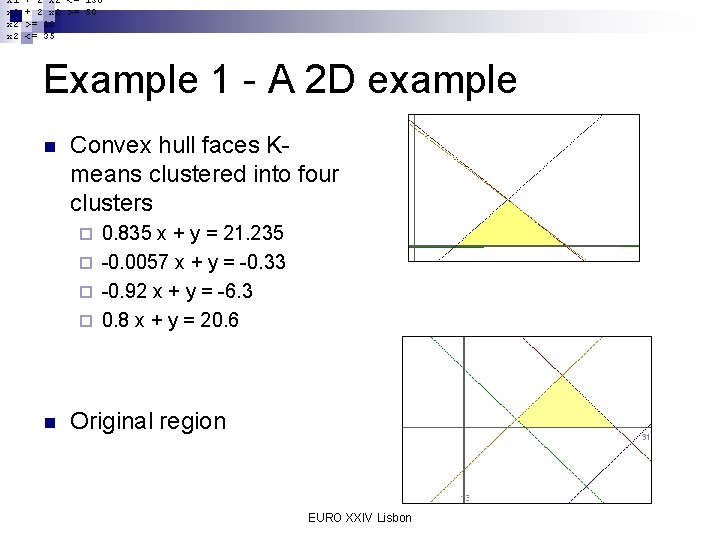x 1 x 2 + 2 x 2 <= 130 + 2 x 2 >= 50 >= 10 <= 35 Example 1 - A 2 D example n Convex hull faces Kmeans clustered into four clusters 0. 835 x + y = 21. 235 ¨ -0. 0057 x + y = -0. 33 ¨ -0. 92 x + y = -6. 3 ¨ 0. 8 x + y = 20. 6 ¨ n Original region EURO XXIV Lisbon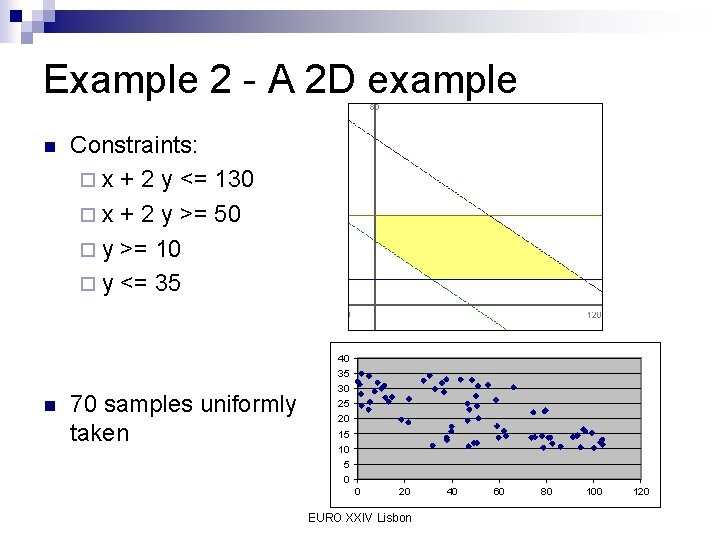Example 2 - A 2 D example n Constraints: ¨ x + 2 y <= 130 ¨ x + 2 y >= 50 ¨ y >= 10 ¨ y <= 35 40 35 n 70 samples uniformly taken 30 25 20 15 10 5 0 0 20 EURO XXIV Lisbon 40 60 80 100 120Example 2 - A 2 D example n n Convex Hull – 14 facets Convex hull faces Kmeans clustered into four clusters EURO XXIV LisbonExample 3 - A 5 D example n Constraints ¨ x 1 + x 2 + x 3 + x 4 + x 5 <= 800 ¨ x 1 + x 2 + x 3 + x 4 + x 5 >= 500 ¨ x 1 - x 2 - x 3 >= 50 ¨ x 1 - x 2 - x 3 <= 100 ¨ x 4 - x 5 >= 30 ¨ x 4 - x 5 <= 70 n Convex hull – 1918 facets EURO XXIV LisbonConclusions n n A new approach to multi-dimensional generalization of the German Tank problem with convergence time, polynomial in accuracy, is presented. This can be used to estimate constraints in a robust optimization approach and is applicable to a wide variety of applications such as robust optimizations in a supply chain. EURO XXIV LisbonThank you EURO XXIV Lisbon